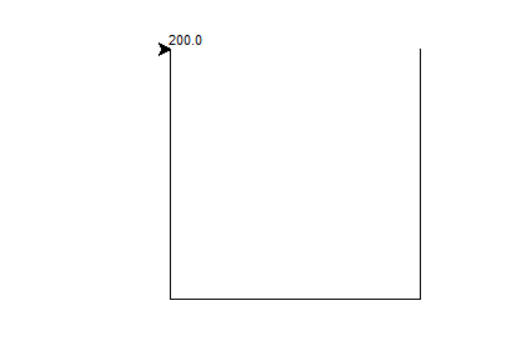• 已知经纬度坐标两点距离，用python表示已知地球上任意两点（lon1, lat1）,（ lon2, lat2）的经纬度坐标，求两点间的距离用haversine表示：1.首先先将经纬度坐标的角度化成弧度（mlon1,mlat1）,(mlon2,mlat2)2....
已知经纬度坐标求两点间距离，用python表示
已知地球上任意两点（lon1, lat1）,（ lon2, lat2）的经纬度坐标，求两点间的距离用haversine表示：
1.首先先将经纬度坐标的角度化成弧度（mlon1,mlat1）,(mlon2,mlat2)
2.利用公式：其中a表示两点维度的差值，即 a = mlat1 - mlat2
其中a表示两点经度的差值，即 b = mlon1 - mlon2
其中r表示地球的半径
用python表示为
def geo_distance(lon1, lat1, lon2, lat2): #地理距离
"""
Calculate the great circle distance between two points
on the earth (specified in decimal degrees)
"""
lon1, lat1, lon2, lat2 = map(radians, map(float, [lon1, lat1, lon2, lat2])) #根据提供的函数对指定序列做映，radians:将角度转换为弧度。
dlon = lon2 - lon1
dlat = lat2 - lat1
a = sin(dlat/2)**2 + cos(lat1) * cos(lat2) * sin(dlon/2)**2
c = 2 * asin(sqrt(a))
r = 6371
return c * r
print(geo_distance(111.5,36.08,121.47,31.23)) #我老家到上海的距离
结果
1067.9651712083848 #单位 km
其实python自带haversine库，可以直接调用，这样就可以不记公式啦！
from haversine import haversine
linfen = (111.5,36.08)
shanghai = (121.47,31.23)
dis = haversine(linfen,shanghai)
print(dis)
结果
1133.5471931316163 #单位 km
可以看出还是有差距的，那那个准确呢？ _用那种方法知道了吧！嘿嘿
展开全文• 类似的需求应该比较多，这里的核心是关于坐标点之间距离计算，之前没用过这个东西，刚好今天有个这样的需求，记录下。 import requests import psycopg2 import pandas as pd from math import radians, ...
背景：有几个门店和大厦，看看这几个门店和大厦旁边有没有快递柜
数据：门店和大厦只有地址数据，快递柜系统有地址数据和经纬度数据
类似的需求应该比较多，这里的核心点是关于两个坐标点之间距离的计算，之前没用过这个东西，刚好今天有个这样的需求，记录下。
import requests
import psycopg2
import pandas as pd
from math import radians, cos, sin, asin, sqrt

#返回地址的坐标
#使用高德地图的api,获取地址的经纬度，注意key用自己的key,这里的key是瞎写的
base = 'http://restapi.amap.com/v3/geocode/geo'
response = requests.get(base, parameters)
return lon,lat

#返回系统所有的深圳快递柜的坐标
def get_cabinet_coordinate():
conn = psycopg2.connect(database="database",
user="root",
host="localhost",
port="443")
cursor = conn.cursor()
sql = "select  * from  t_station where  city_code = 440300"
cursor.execute(sql)
df = pd.DataFrame(cursor.fetchall())
cursor.close()
df = df.iloc[:,[11,3,4,5,6]]
return df

#获取两个坐标点的距离
def get_distance(lon1, lat1, lon2, lat2):
# 将十进制度数转化为弧度
lon1, lat1, lon2, lat2 = map(radians, [lon1, lat1, lon2, lat2])

# haversine公式
dlon = lon2 - lon1
dlat = lat2 - lat1
a = sin(dlat/2)**2 + cos(lat1) * cos(lat2) * sin(dlon/2)**2
c = 2 * asin(sqrt(a))
r = 6371 # 地球平均半径，单位为公里
#print(c * r * 1000)
return c * r * 1000

if __name__ == '__main__':
df = get_cabinet_coordinate()
lst = list()
#print(lon1,lat1)
for i in df.values:
lon2 = i
lat2 = i
distance = get_distance(lon1, lat1, lon2, lat2)
if distance<=3000:
lst.append({
'lon1':lon1,             #经度
'lat1':lat1,             #维度
'sn':i,               #快递柜sn编码
'lon2':i,             #经度
'lat2':i,             #维度
'distance':distance      #快递柜和分析地址距离
})
#print(dic)
df = pd.DataFrame(lst)
df.to_excel(r'C:\Users\James\Desktop\地域数据_距离.xlsx')
print('数据保存成功')
print(df.head())

展开全文• 话不多说，直接上代码 def haversine(lng1, lat1, lng2, lat2):...# 经度1，纬度1，经度2，纬度2 （十进制度数）计算两经纬度坐标点距离 文章摘自 https://blog.csdn.net/vernice/article/details/46581361
话不多说，直接上代码
def haversine(lng1, lat1, lng2, lat2):
lng1, lat1, lng2, lat2 = map(radians, [lng1, lat1, lng2, lat2])
dlon = lng2 - lng1
dlat = lat2 - lat1
a = sin(dlat/2)**2 + cos(lat1) * cos(lat2) * sin(dlon/2)**2
c = 2 * asin(sqrt(a))
r = 6371 # 地球平均半径，单位为公里
return c * r * 1000
# 经度1，纬度1，经度2，纬度2 （十进制度数）计算两经纬度坐标点的距离
文章摘自https://blog.csdn.net/vernice/article/details/46581361
展开全文• 4，write()方法写入计算距离 示例代码如下，IDLE环境下可直接运行 import turtle import math x1,y1 = 100,100 x2,y2 = 100,-100 x3,y3 = -100,-100 x4,y4 = -100,100 turtle.penup() turt...
1，导入turtle模块 2，导入math模块 3，调用math.sqrt()开平方的方法 4，write()方法写入计算的距离 示例代码如下，IDLE环境下可直接运行
import turtle
import math

x1,y1 = 100,100
x2,y2 = 100,-100
x3,y3 = -100,-100
x4,y4 = -100,100

turtle.penup()
turtle.goto(x1,y1)
turtle.pendown()
turtle.goto(x2,y2)
turtle.goto(x3,y3)
turtle.goto(x4,y4)

distance = math.sqrt((x1-x4)**2 + (y1-y4)**2)
turtle.write(distance)


运行结果如下：以上
如有错误，欢迎指正
欢迎粘贴复制 评论转发点赞
轻轻一点 好运连连
展开全文海龟绘图 折线图
• 在WGS84坐标系下，通过Python实现Haversine公式计算两点（经纬度坐标）之间的距离Haversine
• #定义多个坐标 x1,y1 = 100,100 x2,y2 = 100,-100 x3,y3 = -100,-100 x4,y4 = -100,100 #绘制折线 turtle.penup() turtle.goto(x1,y1) turtle.pendown() turtle.goto(x2,y2) turtle.goto(x3,y3) t...
• 两点坐标是(x1, y1)和(x2, y2) 则两点之间的距离公式为 d=√[(x1-x2)²+(y1-y2)²] 注意特例： 当x1=x2时 两点距离为|y1-y2| 当y1=y2时 两点距离为|x1-x2| 中点坐标：midpoint(X,Y) X=(X1+X2)/2 Y...
• ## 用Python如何计算两点间距离

万次阅读 多人点赞 2020-03-02 17:27:36
Python如何计算两点距离？ 第一步，我们先来看一下两点间的距离公式。 两点间距离公式： ？？？这是个啥？ 是不是方法一还能看懂，方法二却一脸懵逼？ 请仔细看注释， 也就是说，根号2还可以写成2的...
• Python两点之间的直线距离(2种实现方法)方法一：#导入math包import math#定义点的函数class Point:def __init__(self,x=0,y=0):self.x=xself.y=ydef getx(self):return self.xdef gety(self):return self.y#定义...
• 本博文源于python基础，旨在探讨python中的lamda表达式应用。内容包含lamda格式/去除列表中偶数/扩大列表中的值/求两点坐标距离函数。列表 lambda
• 计算两点之间的距离日常旁白：本人是一枚生物学的学生，由于对生物信息学特别感兴趣，于是想自学生物信息学（新手莫怪）。了解到生物信息学要有编程基础，尤其是要会一门编程语言，例如：R语言、Python、Perl等，...
• 已知地球上任意两点（lng1，lat1），（lng2， lat2）的经纬度坐标，求两点间的距离可以利用 haversine 公式： 首先先将经纬度坐标的角度化成弧度（rlng1，rlat1），（rlng2，rlat2) 利用如下公式： 其中 a ...haversine geopy
• 今天小编就为大家分享一篇python计算两个地址之间的距离方法，具有很好的参考价值，希望对大家有所帮助。一起跟随小编过来看看吧
• #对于两点坐标为同一点时,返回点与点的距离 if line_point1 == line_point2: point_array = np.array(point ) point1_array = np.array(line_point1) return np.linalg.norm(point_array -point1_array ) #...python
• 种方法，一个是利用python库，另一个利用math函数计算，最后和百度地图测量的做对比 计算结果 447.249799354 446.72072701 447.02 百度地图测量 # 1. Python 库 from geopy.distance import geodesic aa = ...
• 目前各个地图的经纬度坐标还是有差异的，为了开发人员能方便的进行坐标间的转化，顺带分享一个经纬度坐标转换的api， 点击打开链接 还是很不错的，但是好像一天有几千次的限制，不太记得了，管他呢，能用就行。 ...api
• 平常为了得出地理位置上两点的实际距离（譬如北京与杭州之间的实际距离），除了利用经纬度计算两点的空间距离，还需要考虑地形因素。由于之间考虑地形造成误差较大，因此采用微分的办法来解决，简单来说就是将两点...算法
• 比如A点经纬度（110.0123, 23.32435），B点经纬度（129.1344,25.5465），求AB两点之间的距离。我们可以用haversine()函数求出距离结果。Python版本的haversine()如下所示： from math import radians, cos, sin, ...haversine 度数转弧度
• 这种文章本来网上一搜一大把，但有的计算结果是错的，遂找到个计算正确的，记录一下。 参考原文：LBS 球面距离公式 from math import sin, asin, cos, radians, fabs, sqrt EARTH_RADIUS=6371 # 地球平均半径，...lbs 地图
• 已知经纬度坐标两点距离，用python表示 已知地球上任意两点（lon1, lat1）,（ lon2, lat2）的经纬度坐标，求两点间的距离用haversine表示： 1.首先先将经纬度坐标的角度化成弧度（mlon1,mlat1）,(mlon2,mlat2) 2...
• 使用海龟画图 #导入画图 ...#定义多个坐标 x1,y1=100,100 x2,y2=100,-100 x3,y3=-100,-100 x4,y4=-100,100 #绘制折线 turtle.penup() turtle.goto(x1,y12) turtle.pwndown() turtle.goto(x2,y2) ...
• 两点距离 /***** 求两点距离*****/ float getDistance(CvPoint pointO, CvPoint pointA) { float distance; distance = powf((pointO.x - pointA.x), 2) + powf((pointO.y - pointA.y), 2); distance = ...
• 近期在用 OpenCV 时需要... 到直线距离由于项目中得到坐标最容易，因此采用向量法进行所有的数学计算最清晰明了。到直线距离就采用向量法推导。 推导过程： 代码实现：array_longi = np.array([x2-x1, y...
• import math ipt = input('请输入x1,y1,x2,y2，以逗号隔开:') data = ipt.split(',') data = [int(x) for x in ...print(data, ',', data, '与', data, ',', data, '之间的距离为：', result)
• 2、python利用地图两个点的经纬度计算两点距离 　LBS 球面距离公式 美团app筛选“离我最近” 之 地理空间距离计算优化 案例1、2,因为都是用LBS 球面距离公式（haversine公式）结果完全相同， 且与 geopy......

# python计算两点坐标距离python 订阅